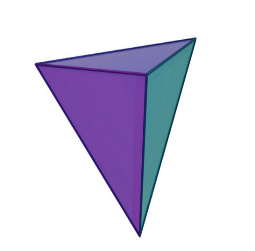# Regular Tetrahedron Formula

Pyramid on a triangular base is a tetrahedron. When a solid is bounded by four triangular faces then it is a tetrahedron. A right tetrahedron is so called when the base of a tetrahedron is an equilateral triangle and other triangular faces are isosceles triangles. When we encounter a tetrahedron that has all its four faces equilateral then it is regular tetrahedron.

#Area of One Face of Regular Tetrahedron Formula:

$\large A=\frac{1}{4}\sqrt{3}a^{2}$

Total Surface Area of Regular Tetrahedron Formula:

$\large A=a^{2}\sqrt{3}$

Slant Height of a Regular Tetrahedron Formula:

$\large a\left(\frac{\sqrt{3}}{2}\right)$

Altitude of a Regular Tetrahedron Formula:

$\large h=\frac{a\sqrt{6}}{3}$

Volume of a Regular Tetrahedron Formula

$\large V=\frac{a^{3}\sqrt{2}}{12}$

### Solved Example

Question: What is the volume of a tetrahedron with sides 10 cm ?

Solution:

Here,
a = 10 cm

Using the formula of Volume of a Regular Tetrahedron:

$$\begin{array}{l}V=\frac{a^{3}\sqrt{2}}{12}\end{array}$$
$$\begin{array}{l}=\frac{10^{3}\sqrt{2}}{12}\end{array}$$
$$\begin{array}{l}=117.85\;cm^{3}\end{array}$$